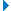# Your grades please for Spurs v Chelsea (H) - (04/02/21)

Text Block:
Please give your honest and fair assessment of the performance of all the players who took part in this game, including playing substitutes. I would prefer that those who complete this function have watched 90 minutes of the game in question.Don't forget to grade those players mentioned in the second section below.
1Here are the first ten team members:-

1 = 1 , 2 = 2 , 3 = 3 , 4 = 4 , 5 = 5 , 6 = 6 , 7 = 7 , 8 = 8 , 9 = 9 , 10 = 10
Answer
 1 2 3 4 5 6 7 8 9 10
Number of
Responses
Rating
Score*
Lloris 149 6.2
Aurier 149 4.9
Alderweireld 150 5.3
Dier 150 3.5
Davies 150 4.2
Sissoko 150 4.1
Hojbjerg 149 5.3
Bergwijn 149 4.1
Ndombele 150 4.7
Son 149 4.0
*The Rating Score is the weighted average calculated by dividing the sum of all weighted ratings by the number of total responses.Show Details
2Don't forget to grade these players too! Note that up to five substitutes are allowed for the remainder of this season, but they have to be made on no more than three occasions.

1 = 1 , 2 = 2 , 3 = 3 , 4 = 4 , 5 = 5 , 6 = 6 , 7 = 7 , 8 = 8 , 9 = 9 , 10 = 10
Answer
 1 2 3 4 5 6 7 8 9 10
Number of
Responses
Rating
Score*
Vinicius 147 4.1
Lamela 147 6.0
Lucas Moura 146 5.0
*The Rating Score is the weighted average calculated by dividing the sum of all weighted ratings by the number of total responses.Show Details
3I would also like you to give marks out of 10 in the following skill areas:-

1 = 1 , 2 = 2 , 3 = 3 , 4 = 4 , 5 = 5 , 6 = 6 , 7 = 7 , 8 = 8 , 9 = 9 , 10 = 10
Answer
 1 2 3 4 5 6 7 8 9 10
Number of
Responses
Rating
Score*
SKILL 140 3.5
PLUCK (EFFORT) 141 3.5
UNFORCED ERRORS (The less errors, the better the mark) 140 3.2
RESOLVE (Defensive ability) 140 3.8
SCORE RATIO (Goals scored in relation to shots) 140 2.7
*The Rating Score is the weighted average calculated by dividing the sum of all weighted ratings by the number of total responses.Show Details
4
Now please grade Jose Mourinho on three areas - Team Selection, Tactics, and Substitutions

1 = 1 , 2 = 2 , 3 = 3 , 4 = 4 , 5 = 5 , 6 = 6 , 7 = 7 , 8 = 8 , 9 = 9 , 10 = 10
Answer
 1 2 3 4 5 6 7 8 9 10
Number of
Responses
Rating
Score*
Team Selection 147 4.4
Tactics 146 2.2
Substitutions 147 4.1
*The Rating Score is the weighted average calculated by dividing the sum of all weighted ratings by the number of total responses.Show Details
5Finally, here's a chance for you to give a single mark out of ten for the performance of the officials, led by referee Andre Marriner.  (VAR has to be considered part of your considerations).

1 = 1 , 2 = 2 , 3 = 3 , 4 = 4 , 5 = 5 , 6 = 6 , 7 = 7 , 8 = 8 , 9 = 9 , 10 = 10
 1 2 3 4 5 6 7 8 9 10
Number of
Responses
Rating
Score*
142 5.0
*The Rating Score is the weighted average calculated by dividing the sum of all weighted ratings by the number of total responses.Show Details